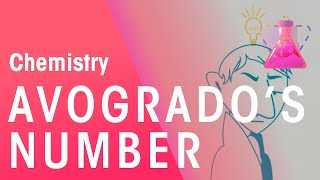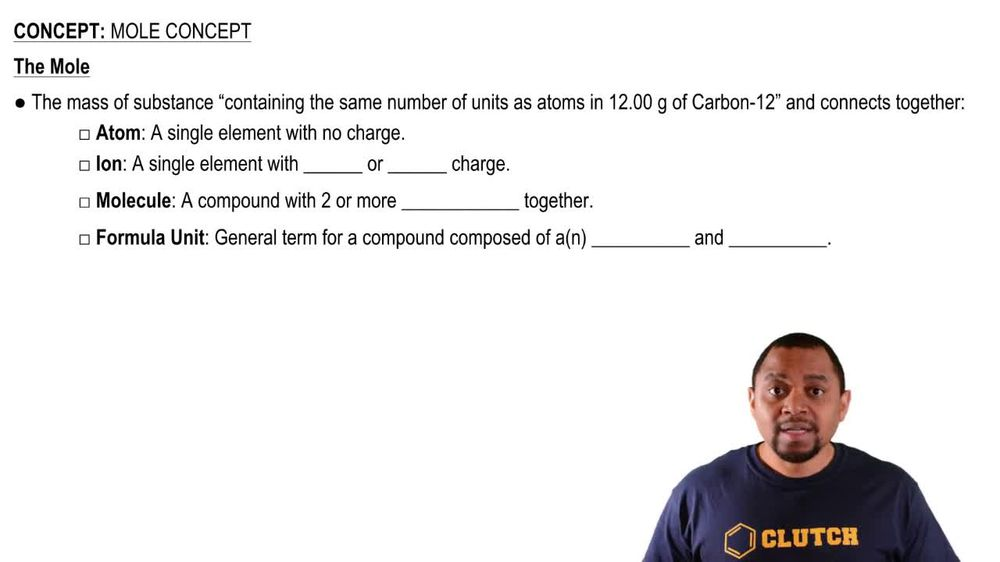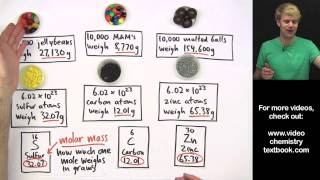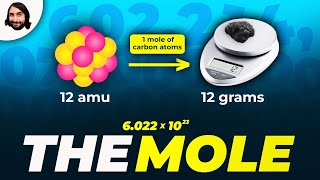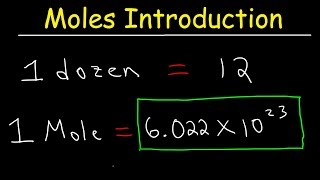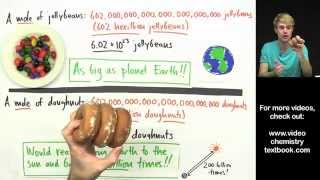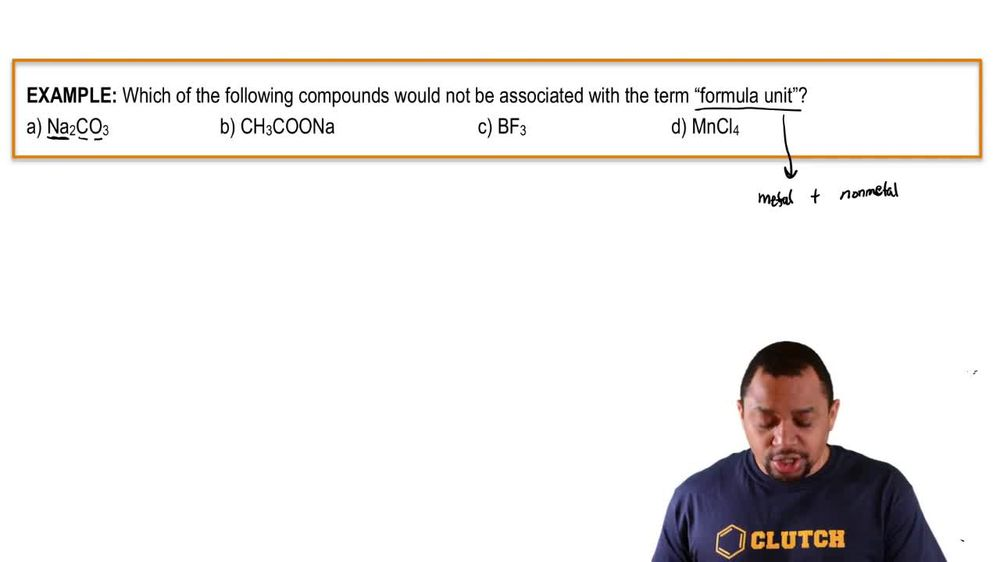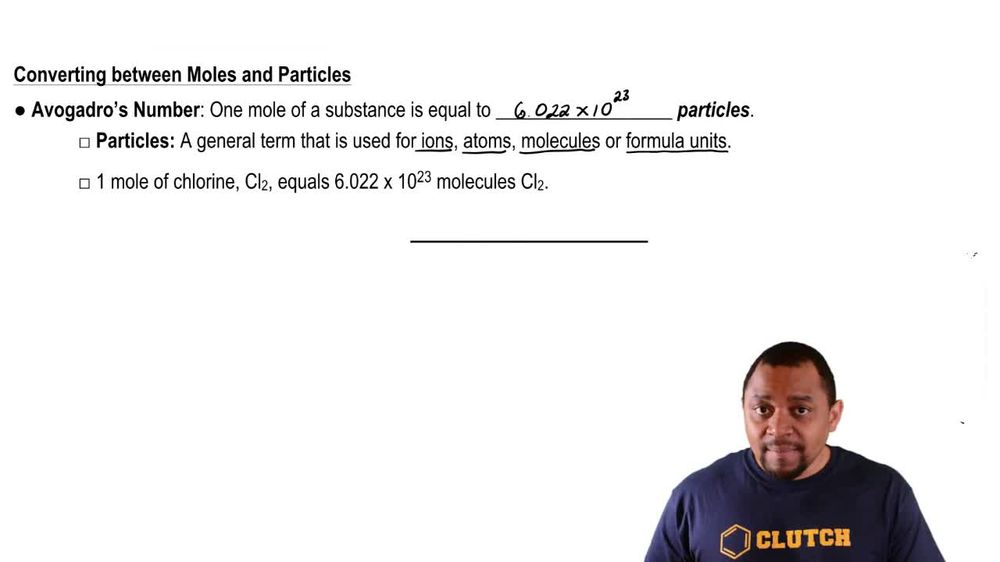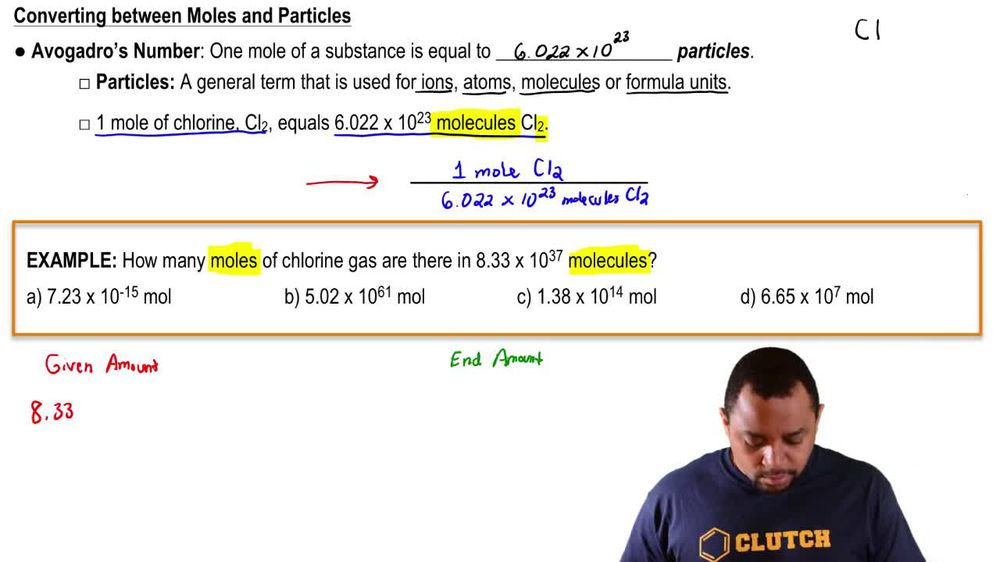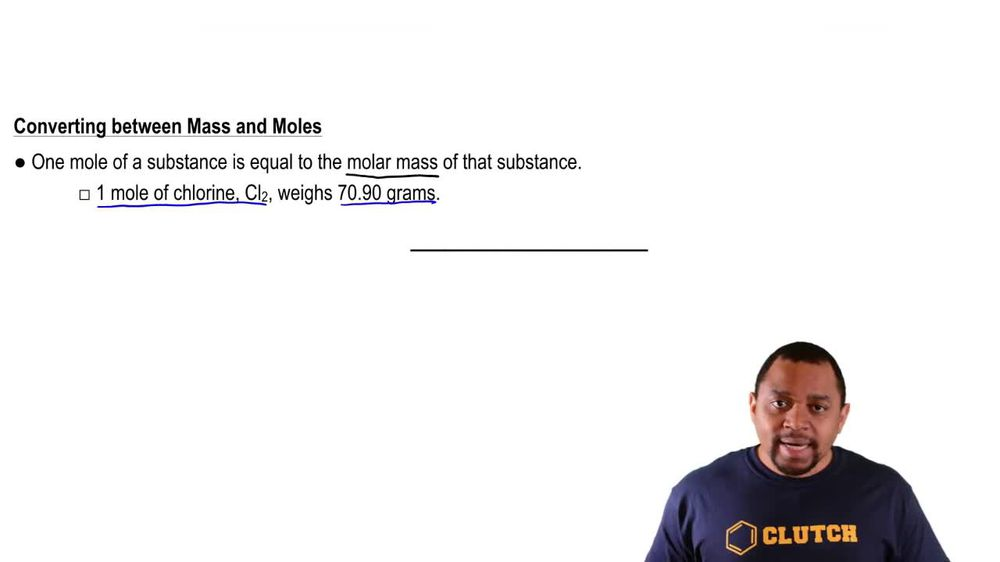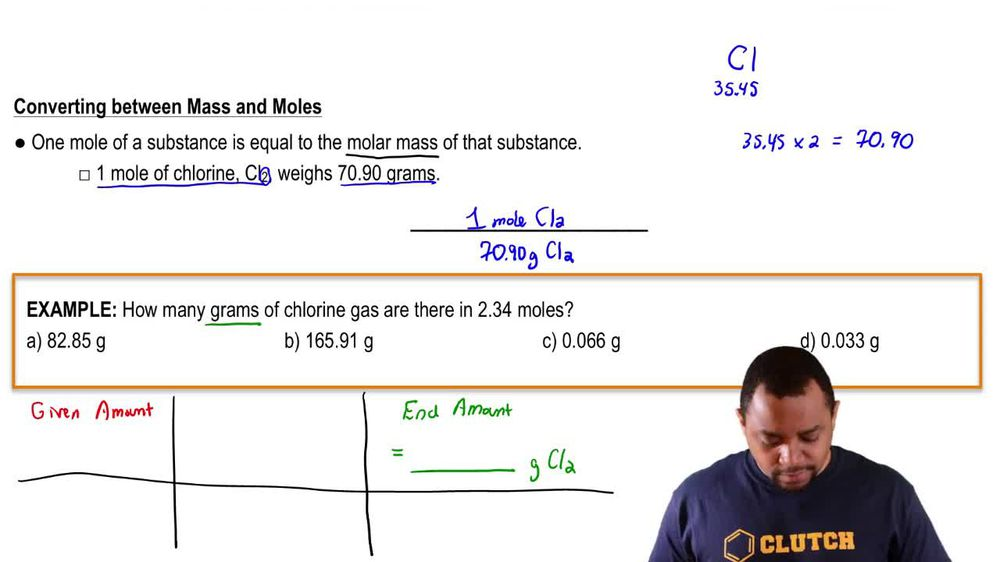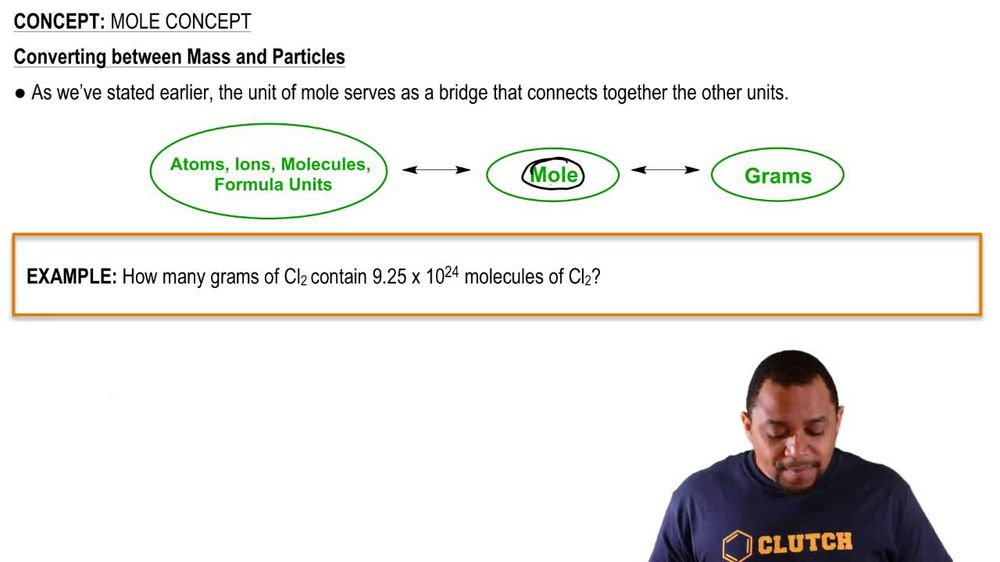Start typing, then use the up and down arrows to select an option from the list.
1. 2. Atoms & Elements2. Mole Concept
Problem

# Calculate the following quantities: (d) number of N atoms in 0.410 mol NH3

Relevant Solution1m
Play a video:
Hello everyone in this question, we asked calculate the number of carbon atoms in 3.21 moles of C six H three BR three. They were given 3.21 moles of C six H three, we are three. And in order to determine the number of carbon atoms, we need to first determine the number of moles of carbon. So in one mole of C six H three we are three, We have six moles of carbon. And in one mole of carbon We have avocados number, which is 6.0-2 Times 10 to Adams of carbon. This is going to give us 1.2, I was 10 to 25 Adams of carbon that is going to be d Thanks for watching my video and I hope it was helpful.